# 清华开源Jittor-首个国内高校自研深度学习框架，

Jittor 官网：https://cg.cs.tsinghua.edu.cn/jittor/

• 易用且可定制：只需要数行代码，就可定义新的算子和模型。
• 实现与优化分离：可以通过前端接口专注于实现，而实现自动被后端优化。
• 所有都是即时的：Jittor 的所有代码都是即时编译并且运行的，包括 Jittor 本身。用户可以随时对 Jittor 的所有代码进行修改，并且动态运行。

Jittor 作为全新的深度学习框架，有几大新特性值得关注。首先，Jittor 采用了元算子的概念，将各种基本计算定义为元算子，并通过结合不同元算子，实现深度学习中的各项算子功能。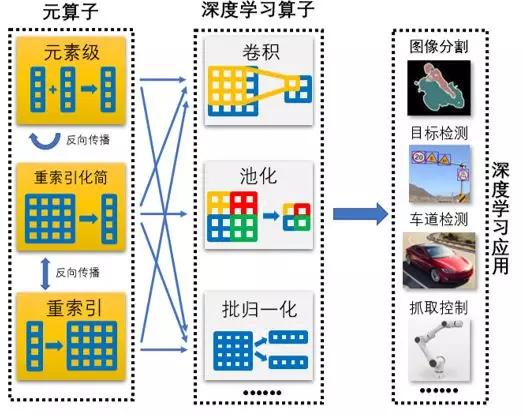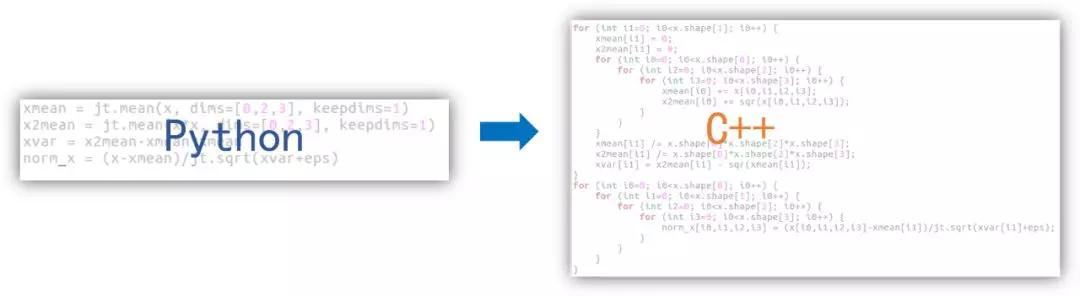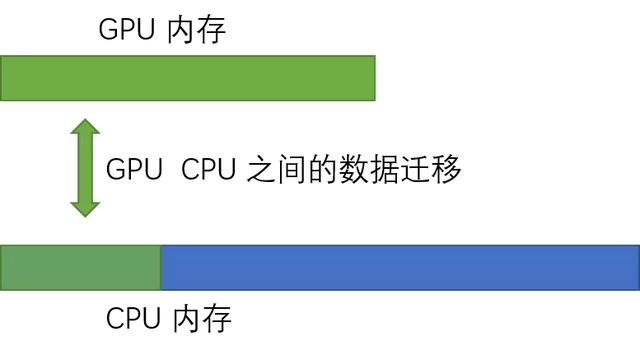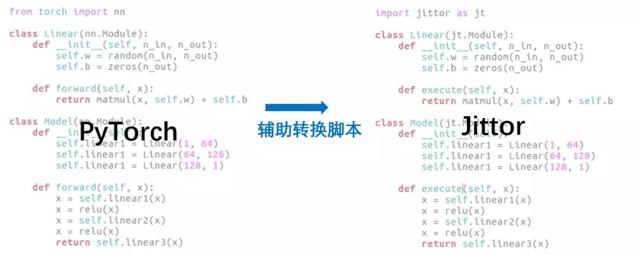Jittor 的性能如何，官方也提供了测评结果进行参考。目前 ResNet、VGG、SSD、DeepLab、LSGAN 等多个网络模型已经在 Jittor 平台实现，可供用户使用。与同类型框架相比，Jittor 在收敛精度一致情况下，推理速度取得了 10%-50% 的性能提升。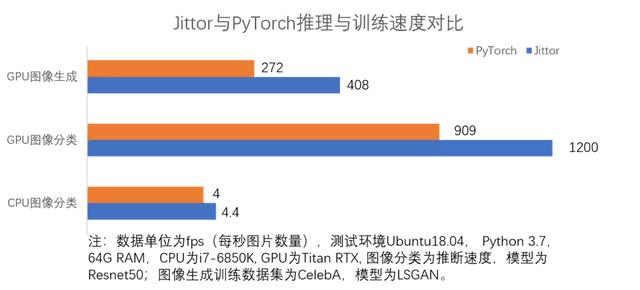Jittor 前端语言为 Python。前端使用模块化的设计，类似于 PyTorch，Keras，后端则使用高性能语言编写，如 CUDA，C++。Jittor 官网目前已提供了示例代码，我们可以来分析一下。

import jittor as jt
from jittor import Module
from jittor import nn
class Model(Module):
def __init__(self):
self.layer1 = nn.Linear(1, 10)
self.relu = nn.Relu()
self.layer2 = nn.Linear(10, 1)
def execute (self,x) :
x = self.layer1(x)
x = self.relu(x)
x = self.layer2(x)
return x
def get_data(n): # generate random data for training test.
for i in range(n):
x = np.random.rand(batch_size, 1)
y = x*x
yield jt.float32(x), jt.float32(y)
model = Model()
learning_rate = 0.1
optim = nn.SGD(model.parameters(), learning_rate)
for i,(x,y) in enumerate(get_data(n)):
pred_y = model(x)
loss = ((pred_y - y)**2)
loss_mean = loss.mean()
optim.step (loss_mean)
print(f"step {i}, loss = {loss_mean.data.sum()}")Jittor 项目的主要研发主力为实验室的梁盾、杨国烨、杨国炜和周文洋等等博士生，此外该项目也得到了清华-腾讯联合实验室的资助和支持。